deutsch     english    français     Print

## 11.3 BUGS & DEBUGGING### INTRODUCTION

 There is no absolute perfection. You can assume that practically every software has its faults. Instead of calling these mistakes, in programming we call them bugs. These manifest themselves when, for example, the program produces incorrect results under certain circumstances or when it even crashes. Troubleshooting, called debugging, is therefore almost as important as writing code. It is understood, of course, that anyone who writes code should do their best to avoid bugs. Knowing that bugs are everywhere, you should be careful and program defensively. If you are not sure if an algorithm or a piece of the code is correct, it is better if you engage with it especially intensely instead of rushing through it as quickly as possible or putting it off for later. Nowadays there is a lot of software whose main function is dealing with large sums of money or even human lives that are at stake. As a programmer of such mission critical software, you need to be absolutely sure and take the responsibility that every line of code works correctly. Quick hacks and the principle of trial and error are not appropriate there. The crucial objective of developing algorithms and large software systems is to produce programs that are as error-free as possible. There are many approaches for this: You could try to prove the correctness of programs in a mathematically precise manner without using the computer, although this can only be done with short programs. Another possibility that exists is to limit the syntax of the programming language so that the programmer can definitely not make certain mistakes. The most important example is the elimination of pointer variables (pointers) that may refer to undefined or wrong objects when not used properly. This is why there are programming languages with many restrictions of this kind, and others that give the programmer a considerable amount of freedom and which are therefore less secure. Python belongs to the class of more liberal programming languages and is based on the motto: "We are all adults and decide for ourselves what we do and what we shouldn't." or "After all, we are all consenting adults here". An important principle for creating software as error-free as possible is Design by Contract (DbC). It goes back to Bertrand Meyer at ETH Zürich, the father of the programming language Eiffel. Meyer views software as an agreement between the programmer A and the user B, where  B could again be a programmer who uses the modules and libraries designed by A. In a contract, A determines under which conditions (preconditions) its modules will provide the correct results and then describes it exactly (postconditions). In other words: User B complies with the preconditions so that they have the guarantee from A that they will receive a result in accordance with the postconditions. B does not need to know the implementation of the modules and A can change this at any time without affecting B.

### ASSERTIONS

Since A, the producer of the module, certainly does not really trust the user B, A incorporates tests into their software, which check the required preconditions. These are called assertions. If the assertions are not observed, the program usually terminates with an error message. (It is also conceivable that the error is only caught without the program terminating.) In this case, the following principle of software development comes into play:

Forcing a program termination with a clear description of the possible cause (an error message) is better than an incorrect result.

 As programmer A, you write a function sinc(x) (sinus cardinalis) in your example, which plays an important role in signal processing. It reads as follows:As user B, you want to graphically represent the function values in the range from x = -20 to x = 20.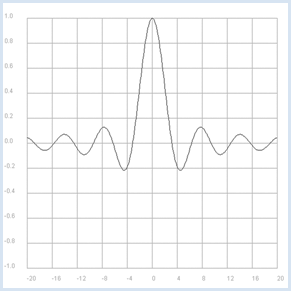```from gpanel import *
from math import pi, sin

def sinc(x):
y = sin(x) / x
return y

makeGPanel(-24, 24, -1.2, 1.2)
drawGrid(-20, 20, -1.0, 1, "darkgray")
title("Sinus Cardinalis: y = sin(x) / x")

x = -20
dx = 0.1
while x <= 20:
y = sinc(x)
if x == -20:
move(x, y)
else:
draw(x, y)
x += dx
```
Highlight program code (Ctrl+C copy, Ctrl+V paste)

 At first glance there do not seem to be any problems, but then if you (as the user B) change the increment of x to 1, there is a bad crash.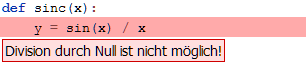A way to solve the problem is for A to require the precondition that x is not 0, and output an assertion that describes the error.
```from gpanel import *
from math import pi, sin

def sinc(x):
assert x == 0, "Error in sinc(x). x = 0 not allowed"
y = sin(x) / x
return y

makeGPanel(-24, 24, -1.2, 1.2)
drawGrid(-20, 20, -1.0, 1, "darkgray")
title("Sinus Cardinalis: y = sin(x) / x")

x = -20
dx = 1
while x <= 20:
y = sinc(x)
if x == -20:
move(x, y)
else:
draw(x, y)
x += dx
```
Highlight program code (Ctrl+C copy, Ctrl+V paste)

 The error message is now much better, since it says exactly where the error occurs. It would be even better if A wrote the function so that the limit value 1 of the function is returned when x = 0.
```from gpanel import *
from math import pi, sin

def sinc(x):
if x == 0:
return 1.0
y = sin(x) / x
return y

makeGPanel(-24, 24, -1.2, 1.2)
drawGrid(-20, 20, -1.0, 1, "darkgray")
title("Sinus Cardinalis: y = sin(x) / x")

x = -20
dx = 1
while x <= 20:
y = sinc(x)
if x == -20:
move(x, y)
else:
draw(x, y)
x += dx
```
Highlight program code (Ctrl+C copy, Ctrl+V paste)

This code, however, contradicts the basic rule that equality tests with floats are dangerous due to possible rounding errors. Even better would be to test it with an epsilon boundary:

```def sinc(x):
epsilon = 1e-100
if abs(x) < epsilon:
return 1.0
```

Your function sinc(x) is still not secure, however, since another precondition should be that x is a number. If you, for instance, call sinc(x) with the value x = "python", it results again in a nasty runtime error.

```from math import sin

def sinc(x):
y = sin(x) / x
return y

print sinc("python")
```
Highlight program code (Ctrl+C copy, Ctrl+V paste)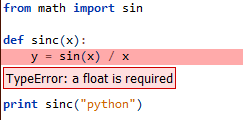This shows the advantage of programming languages with variable declarations, as this error would be already discovered as a syntax error before the execution of the program.

### WRITING OUT DEBUGGING INFORMATION

 Successful programmers are known for their ability to eliminate errors quickly [more... There is a whole software development based on the Test Driven Development (TDD)]. Since we all learn from our mistakes, consider the following program that should exchange the values of two variables. There is a bug, because it outputs 2,2. ```def exchange(x, y): y = x x = y return x, y a = 2 b = 3 a, b = exchange(a, b) print a, b ``` Highlight program code (Ctrl+C copy, Ctrl+V paste) A well-known and simple strategy for finding bugs is to write out the current values of certain variables to the console: ```def exchange(x, y): print "exchange() with params", x, y y = x x = y print "exchange() returning", x, y return x, y a = 2 b = 3 a, b = exchange(a, b) print a, b ``` Highlight program code (Ctrl+C copy, Ctrl+V paste) Hence, it becomes obvious where the error occurred and how you can easily fix it. ```def exchange(x, y): print "exchange() with params", x, y temp = y y = x x = temp print "exchange() returning", x, y return x, y a = 2 b = 3 a, b = exchange(a, b) print a, b ``` Highlight program code (Ctrl+C copy, Ctrl+V paste) Now that the error is fixed, the additional debug lines in the code are unnecessary. Instead of deleting them, you can simply comment them out using the # symbol since you might need them again later. ```def exchange(x, y): # print "exchange() with params", x, y temp = y y = x x = temp # print "exchange() returning", x, y return x, y a = 2 b = 3 a, b = exchange(a, b) print a, b ``` Highlight program code (Ctrl+C copy, Ctrl+V paste) It is smart to use debug flags with which you can activate or deactivate debugging information in different places. ```def exchange(x, y): if debug: print "exchange() with params", x, y temp = y y = x x = temp if debug: print "exchange() returning", x, y return x, y debug = False a = 2 b = 3 a, b = exchange(a, b) print a, b ``` Highlight program code (Ctrl+C copy, Ctrl+V paste) In Python, as you probably already know, you can swap variable values in an elegant way without using an auxiliary variable. For this, you can use the automatic packing/unpacking of tuples. ```def exchange(x, y): x, y = y, x return x, y a = 2 b = 3 a, b = exchange(a, b) print a, b ``` Highlight program code (Ctrl+C copy, Ctrl+V paste)

### USING THE DEBUGGER

Debuggers are important tools for developing large program systems. You can run a program slowly with them, and even run it step by step. So to speak, the enormous execution speed of the computer is adjusted to the limited human cognitive ability. A confortable debugger is ncluded in TigerJython which can help you to better understand the program sequence in a correct program. You are now going to analyze the above defective program with the debugger.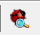After you have taken it to the editor, click on the debugger button.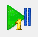You can run the program step by step with the single step button and observe the variables in the debugger window. After clicking 8 times you see that both variables x and y have the value 2 before returning from the function exchange()..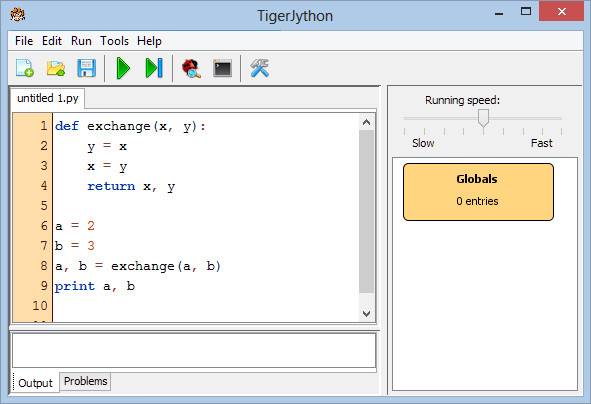If you now run the program with the fixed bug, you can observe how the variables are assigned to one another in exchange(), which finally leads to the correct results. The short life span of the local variables x and y are clearly visible, as opposed to the global variables a and b.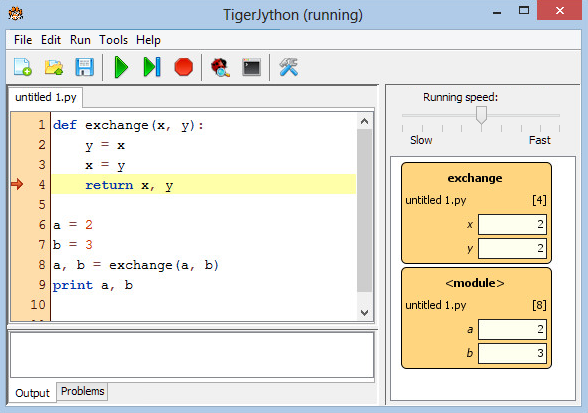You can also set breakpoints in the program so that you do not have to run through tedious non-critical parts of the program in the single step mode. To do this, click on the far left of the line number column. A flag icon appears which marks the breakpoint. When you press the Run button, the program runs up to this point and then stops.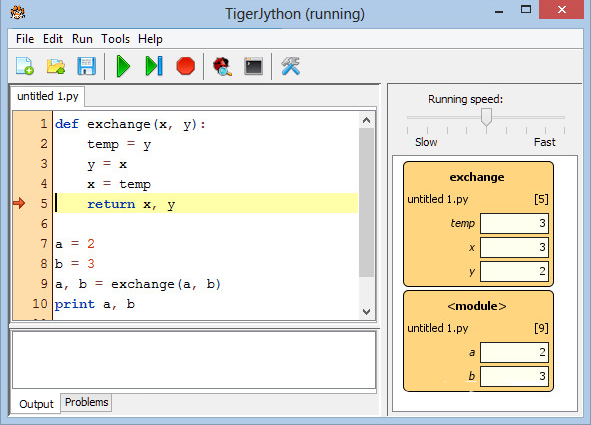This gives you the chance to inspect the current state of the variables. By clicking on the Run button again you can continue to run through the program, or you can investigate it gradually with the single step button. You can also set multiple breakpoints. In order to delete a breakpoint, simply click on the flag icon.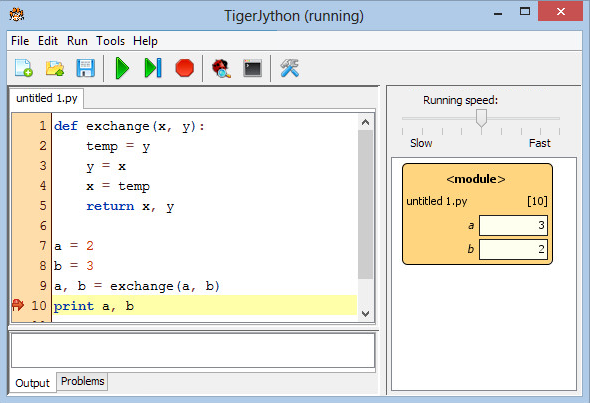### CATCHING ERRORS WITH EXCEPTIONS

 The method of using exceptions to catch errors is classic. To do this, you put the critical program code in a try block. If the error occurs, the block is abandoned and the program continues to run in the except block, where you can react to the error in an appropriate way. In the worst case, you can stop the program execution by calling sys.exit(). You can, for example, catch the error if the parameter in sinc(x) is not of a numeric data type. ```from sys import exit from math import sin def sinc(x): try: if x == 0: return 1.0 y = sin(x) / x except TypeError: print "Error in sinc(x). x =", x, "is not a number" exit() return y print sinc("python") ``` Highlight program code (Ctrl+C copy, Ctrl+V paste) The postcondition for sinc(x) could also mean that the returned value is None if the parameter has an incorrect type. It is then up to the user to deal with this error accordingly. ```from math import sin def sinc(x): try: if x == 0: return 1.0 y = sin(x) / x except TypeError: return None return y y = sinc("python") if y == None: print "Illegal call" else: print y ``` Highlight program code (Ctrl+C copy, Ctrl+V paste)